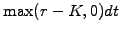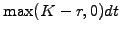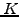SYLLABUS  Previous: 5.2.1 Vanilla swaps  Up: 5.2 Credit derivatives  Next: 5.3 Methods for bonds

### 5.2.2 Cap-/floorlets

[ SLIDE swap rate - experiments || VIDEO login]

The cap-floor parity relation (2.2.4#eq.3) shows that both share many features with swaps. The contract is again decomposed into elementary intervals and the valuation of every caplet or floorlets is carried out backwards starting from zero, since a finite time has to pass to accumulate interest rate earnings. The incremental change reflects the difference between the spot and the cap-/floor rates; contrary to swaps, this difference can never become negative, since caps and floors do not carry any obligation. Using the Vasicek model, the contract can therefore be viewed as a bond paying a continuously compounded annual coupon offor caplets andfor floorlets. By analogy with the swap rate, define the

• the interest cap-/floor rate (or `StrikePrice`), expressed as the relative annual return above /below which the contract pays the rate difference, e.g. 0.04 for a cap rate of 4%.

The VMARKET applet below calculates the value of a caplet with a cap rate of 8%, as a function of the spot rate and an increasing time to the maturity.

 Virtual market experiments: parameters of a caplet Verify that holding a Caplet and shorting a Floorlet in a portfolio results in the same payoff as the swap that can be reproduced by selecting a IRateSwap. Press Display to discuss in detail the similarities and the differences you notice in a comparison with the payoff from call / put options on shares. Why is the payoff deep ``in-the-money'' here curved? Set Volatility=0.05 and more to study how uncertainties affect the prices. Examine the effect of drifts in the forecasted rates by changing parameters such as MktPriceRsk, MeanRevTarg, MeanRevVelo, UserDouble.

The same models used to forecast drifts in the interest rate can also be used here, but the volatility should be modified to reflect the uncertainty in the interest earnings (exercise 5.07).

SYLLABUS  Previous: 5.2.1 Vanilla swaps  Up: 5.2 Credit derivatives  Next: 5.3 Methods for bonds